# Virial decomposition

(diff) ← Older revision | Latest revision (diff) | Newer revision → (diff)

virial series

The series on the right-hand side of the equation of state of a gas: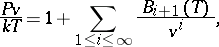whereis the pressure,is the temperature,is the specific volume, andis the Boltzmann constant. The term of the series which contains the-th virial coefficient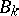describes the deviation of the gas from ideal behaviour due to the interaction in groups ofmolecules.can be expressed in terms of irreducible repeated integrals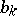: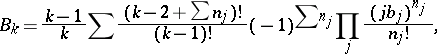summed over all natural numbers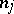,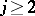, subject to the condition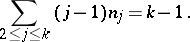In particular,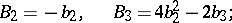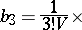where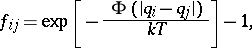is the volume of the gas, the integration extends over the total volume occupied by the gas, andis the interaction potential. There is a rule for writing down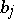for anyin terms of. The expression obtained after simplification is:In practice, only the first few virial coefficients can be calculated.

Power series in, with coefficients expressed in terms of, can be used to represent equilibrium correlation functions forparticles; a corollary of this fact is that the equation of state can be obtained in a simple manner .

There exists a quantum-mechanical analogue of the virial decomposition.

How to Cite This Entry:
Virial decomposition. Encyclopedia of Mathematics. URL: http://encyclopediaofmath.org/index.php?title=Virial_decomposition&oldid=16492
This article was adapted from an original article by I.P. Pavlotskii (originator), which appeared in Encyclopedia of Mathematics - ISBN 1402006098. See original article